# RD Sharma Solutions For Class 7 Maths Exercise 7.4 Chapter 7 Algebraic Expressions

Get free PDF of RD Sharma Solutions for Class 7 Maths Exercise 7.4 of Chapter 7 Algebraic Expressions from the given links. BYJU’S experts have solved the questions present in this exercise, and this will help them more comfortably. This exercise discusses the removal of brackets. In simplifying algebraic expressions, we first remove the grouping symbols by using the following rules:

• If a ‘+’ sign precedes a symbol of grouping symbol may be removed without any change in the sign of the terms
• If a ‘-’ sign precedes a symbol of grouping, the grouping symbol may be removed and the sign of each term is changed.

## Download the PDF of RD Sharma Solutions For Class 7 Maths Chapter 7 – Algebraic Expressions Exercise 7.4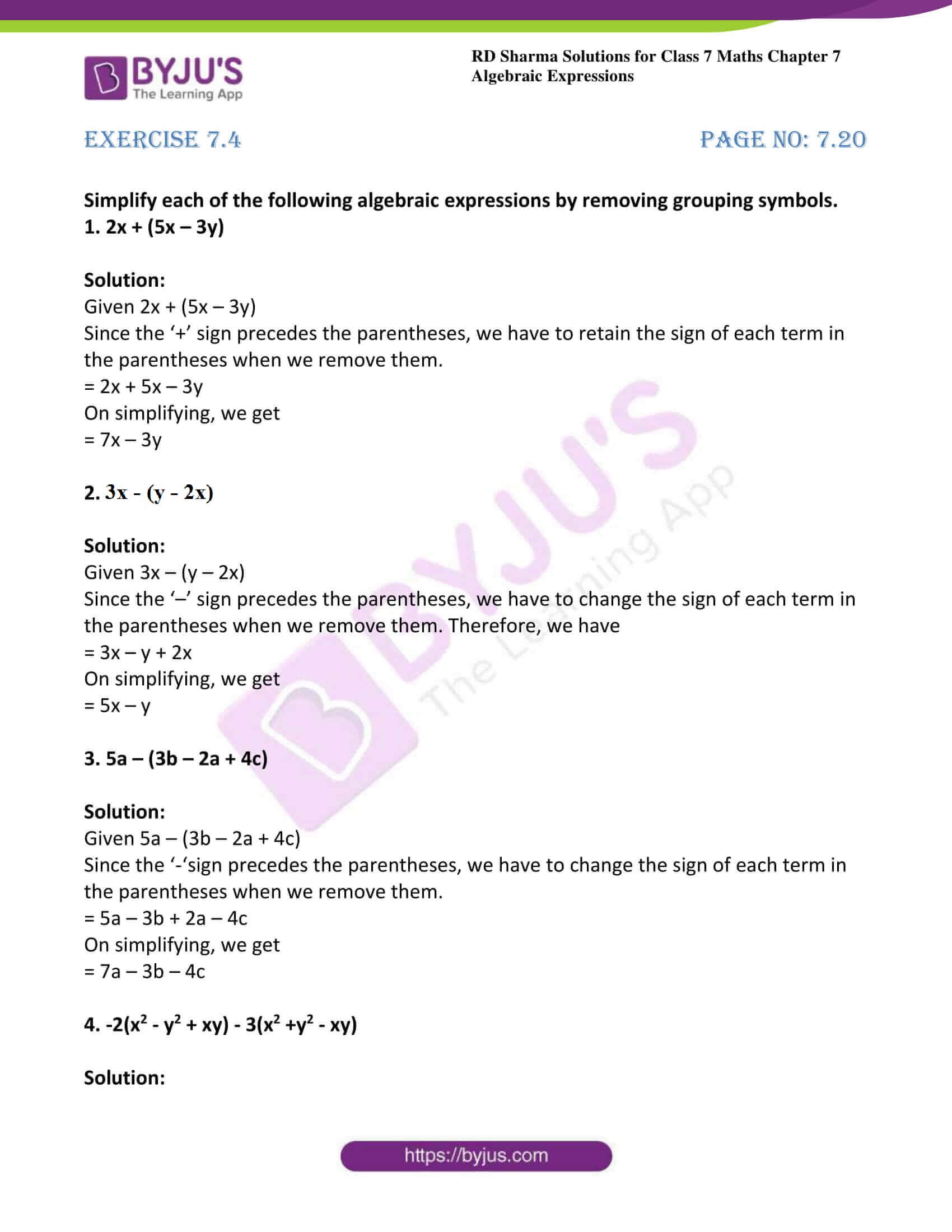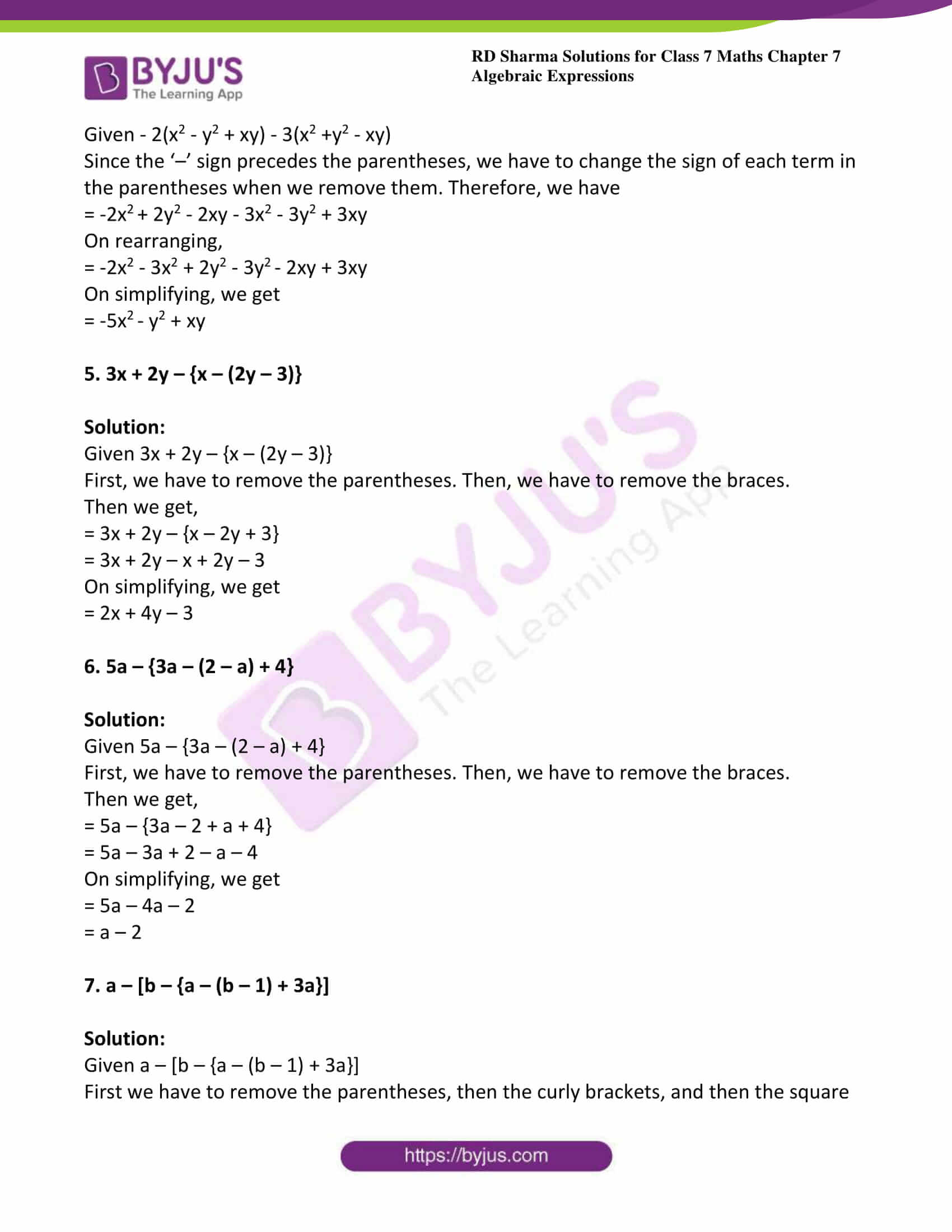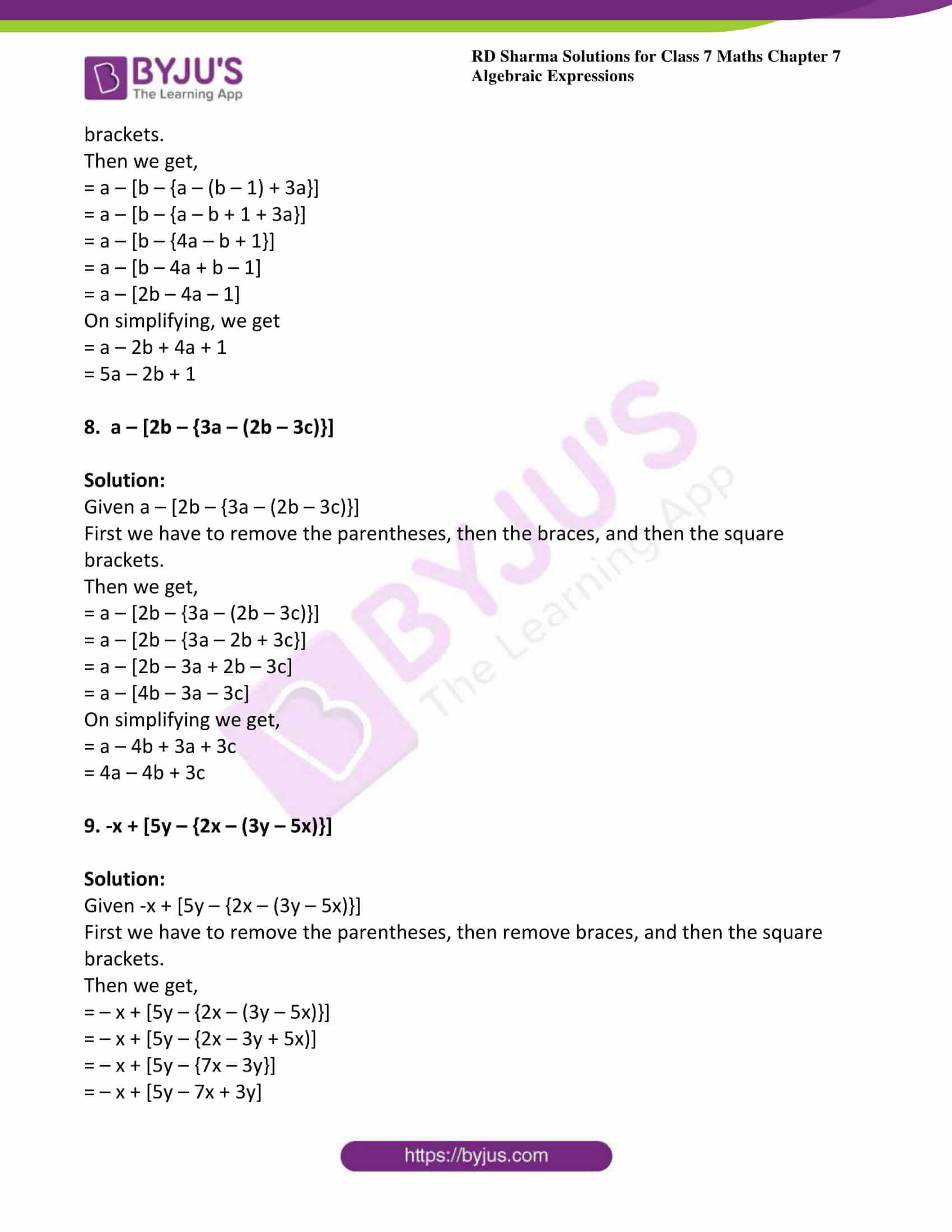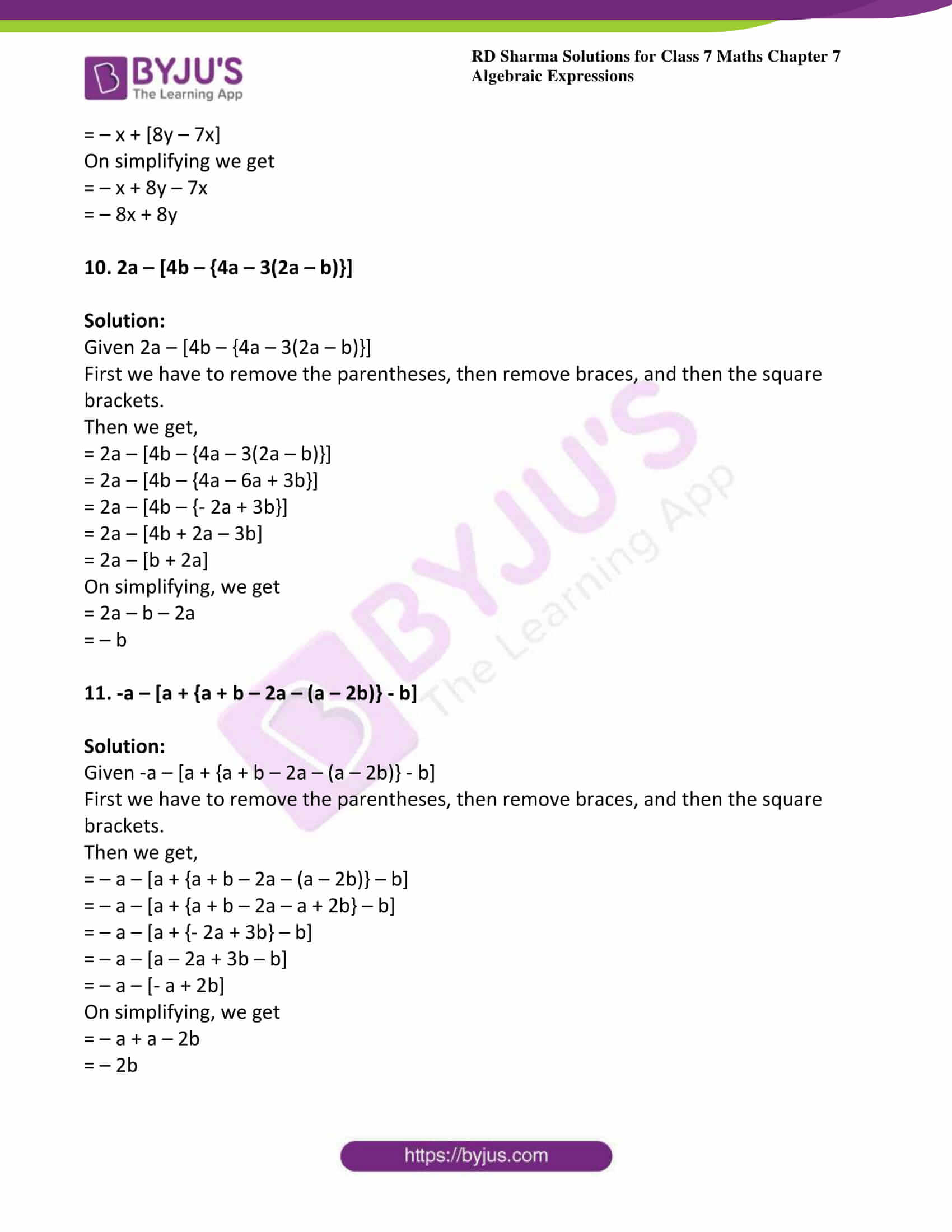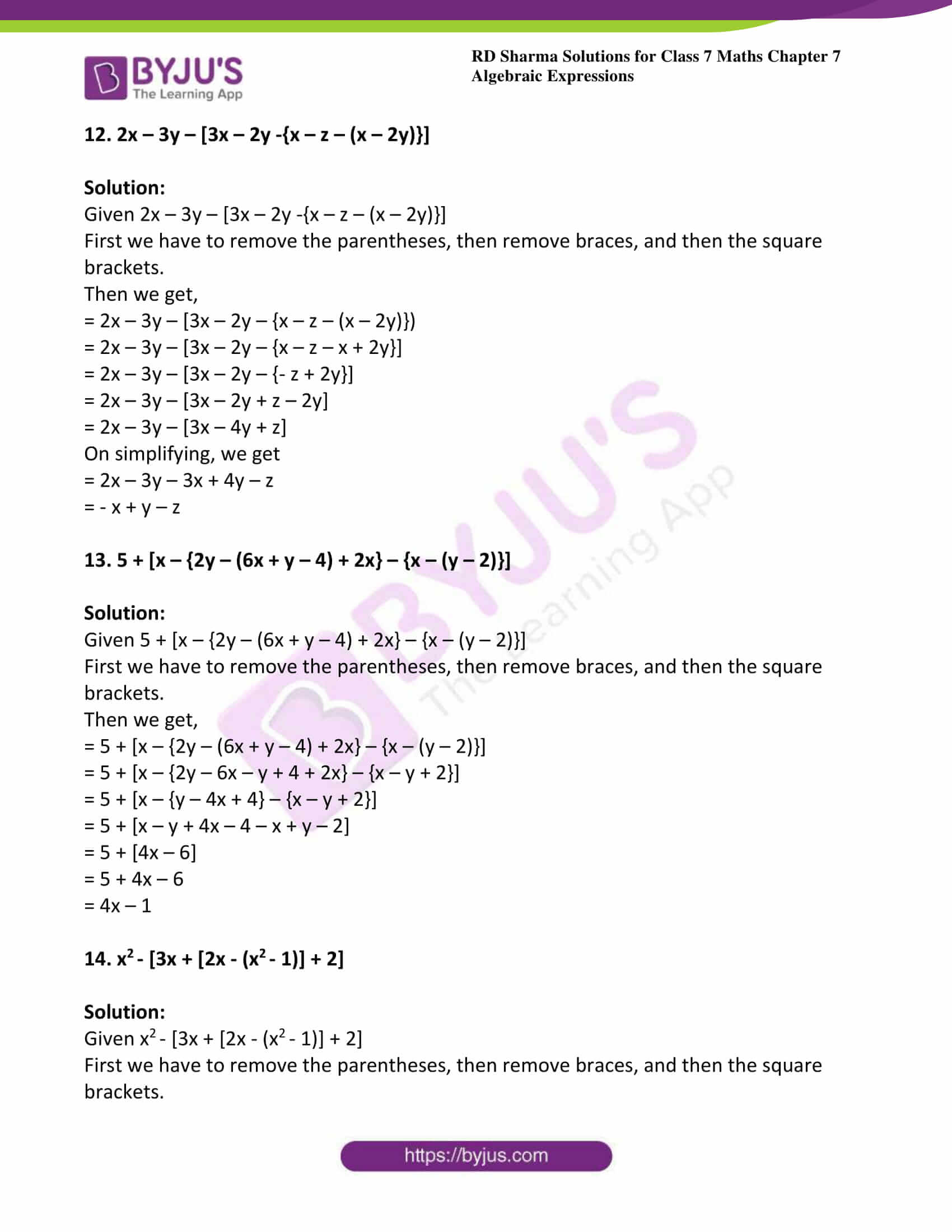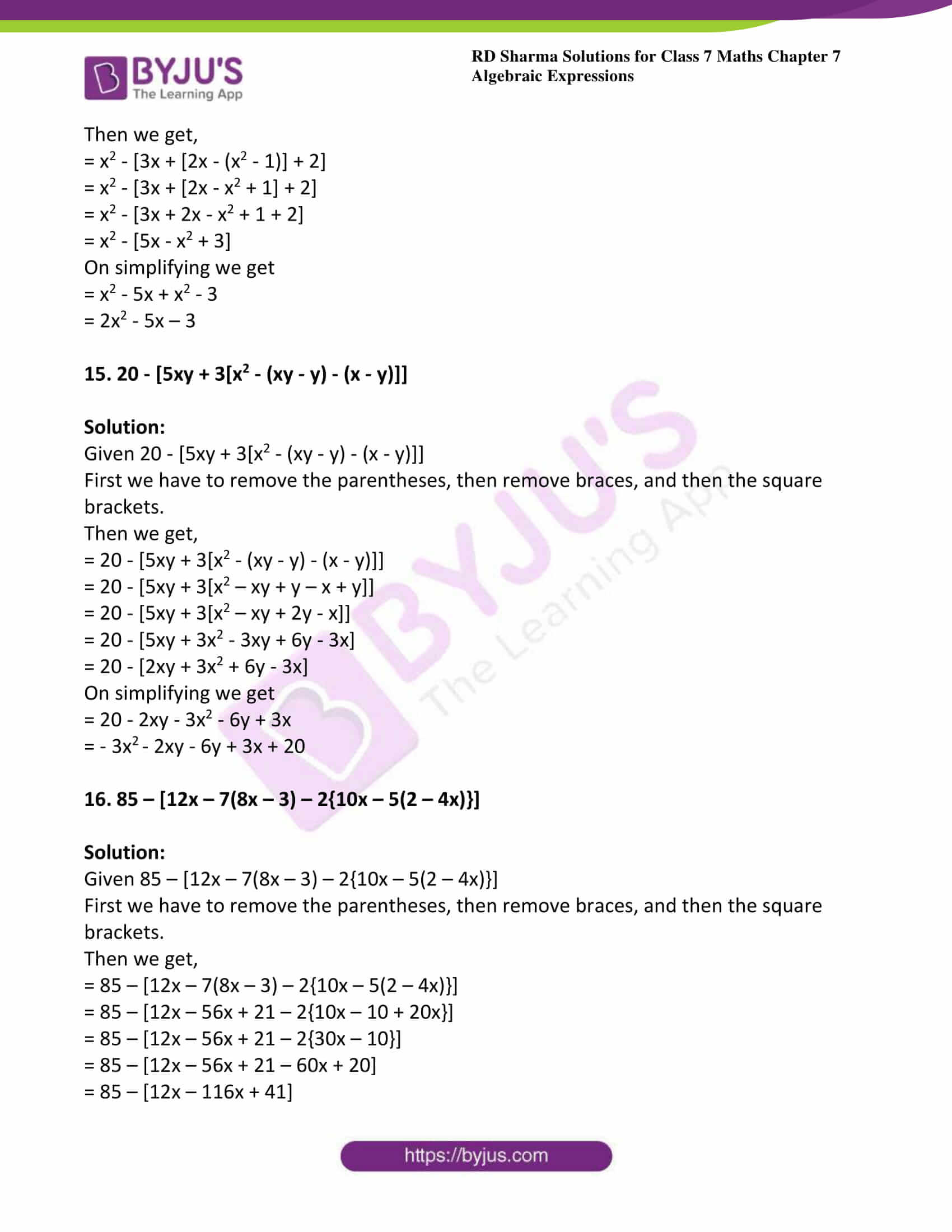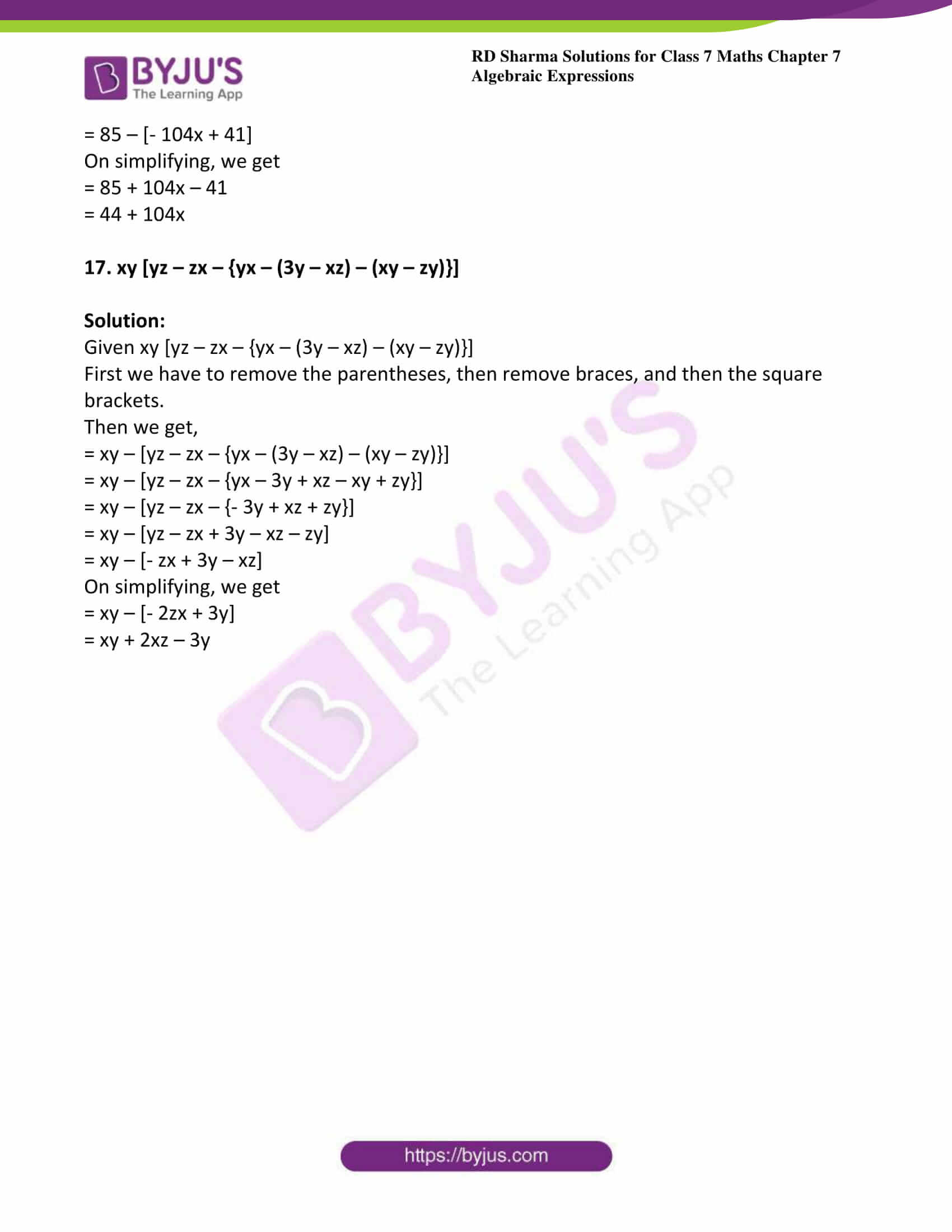### Access answers to Maths RD Sharma Solutions For Class 7 Chapter 7 – Algebraic Expressions Exercise 7.4

Simplify each of the following algebraic expressions by removing grouping symbols.

1. 2x + (5x – 3y)

Solution:

Given 2x + (5x – 3y)

Since the ‘+’ sign precedes the parentheses, we have to retain the sign of each term in the parentheses when we remove them.

= 2x + 5x – 3y

On simplifying, we get

= 7x – 3y

2. 3x – (y – 2x)

Solution:

Given 3x – (y – 2x)

Since the ‘–’ sign precedes the parentheses, we have to change the sign of each term in the parentheses when we remove them. Therefore, we have

= 3x – y + 2x

On simplifying, we get

= 5x – y

3. 5a – (3b – 2a + 4c)

Solution:

Given 5a – (3b – 2a + 4c)

Since the ‘-‘sign precedes the parentheses, we have to change the sign of each term in the parentheses when we remove them.

= 5a – 3b + 2a – 4c

On simplifying, we get

= 7a – 3b – 4c

4. -2(x2 – y2 + xy) – 3(x2 +y2 – xy)

Solution:

Given – 2(x2 – y2 + xy) – 3(x2 +y2 – xy)

Since the ‘–’ sign precedes the parentheses, we have to change the sign of each term in the parentheses when we remove them. Therefore, we have

= -2x+ 2y2 – 2xy – 3x2 – 3y2 + 3xy

On rearranging,

= -2x2 – 3x2 + 2y2 – 3y– 2xy + 3xy

On simplifying, we get

= -5x– y2 + xy

5. 3x + 2y – {x – (2y – 3)}

Solution:

Given 3x + 2y – {x – (2y – 3)}

First, we have to remove the parentheses. Then, we have to remove the braces.

Then we get,

= 3x + 2y – {x – 2y + 3}

= 3x + 2y – x + 2y – 3

On simplifying, we get

= 2x + 4y – 3

6. 5a – {3a – (2 – a) + 4}

Solution:

Given 5a – {3a – (2 – a) + 4}

First, we have to remove the parentheses. Then, we have to remove the braces.

Then we get,

= 5a – {3a – 2 + a + 4}

= 5a – 3a + 2 – a – 4

On simplifying, we get

= 5a – 4a – 2

= a – 2

7. a – [b – {a – (b – 1) + 3a}]

Solution:

Given a – [b – {a – (b – 1) + 3a}]

First we have to remove the parentheses, then the curly brackets, and then the square brackets.

Then we get,

= a – [b – {a – (b – 1) + 3a}]

= a – [b – {a – b + 1 + 3a}]

= a – [b – {4a – b + 1}]

= a – [b – 4a + b – 1]

= a – [2b – 4a – 1]

On simplifying, we get

= a – 2b + 4a + 1

= 5a – 2b + 1

8.  a – [2b – {3a – (2b – 3c)}]

Solution:

Given a – [2b – {3a – (2b – 3c)}]

First we have to remove the parentheses, then the braces, and then the square brackets.

Then we get,

= a – [2b – {3a – (2b – 3c)}]

= a – [2b – {3a – 2b + 3c}]

= a – [2b – 3a + 2b – 3c]

= a – [4b – 3a – 3c]

On simplifying we get,

= a – 4b + 3a + 3c

= 4a – 4b + 3c

9. -x + [5y – {2x – (3y – 5x)}]

Solution:

Given -x + [5y – {2x – (3y – 5x)}]

First we have to remove the parentheses, then remove braces, and then the square brackets.

Then we get,

= – x + [5y – {2x – (3y – 5x)}]

= – x + [5y – {2x – 3y + 5x)]

= – x + [5y – {7x – 3y}]

= – x + [5y – 7x + 3y]

= – x + [8y – 7x]

On simplifying we get

= – x + 8y – 7x

= – 8x + 8y

10. 2a – [4b – {4a – 3(2a – b)}]

Solution:

Given 2a – [4b – {4a – 3(2a – b)}]

First we have to remove the parentheses, then remove braces, and then the square brackets.

Then we get,

= 2a – [4b – {4a – 3(2a – b)}]

= 2a – [4b – {4a – 6a + 3b}]

= 2a – [4b – {- 2a + 3b}]

= 2a – [4b + 2a – 3b]

= 2a – [b + 2a]

On simplifying, we get

= 2a – b – 2a

= – b

11. -a – [a + {a + b – 2a – (a – 2b)} – b]

Solution:

Given -a – [a + {a + b – 2a – (a – 2b)} – b]

First we have to remove the parentheses, then remove braces, and then the square brackets.

Then we get,

= – a – [a + {a + b – 2a – (a – 2b)} – b]

= – a – [a + {a + b – 2a – a + 2b} – b]

= – a – [a + {- 2a + 3b} – b]

= – a – [a – 2a + 3b – b]

= – a – [- a + 2b]

On simplifying, we get

= – a + a – 2b

= – 2b

12. 2x – 3y – [3x – 2y -{x – z – (x – 2y)}]

Solution:

Given 2x – 3y – [3x – 2y -{x – z – (x – 2y)}]

First we have to remove the parentheses, then remove braces, and then the square brackets.

Then we get,

= 2x – 3y – [3x – 2y – {x – z – (x – 2y)})

= 2x – 3y – [3x – 2y – {x – z – x + 2y}]

= 2x – 3y – [3x – 2y – {- z + 2y}]

= 2x – 3y – [3x – 2y + z – 2y]

= 2x – 3y – [3x – 4y + z]

On simplifying, we get

= 2x – 3y – 3x + 4y – z

= – x + y – z

13. 5 + [x – {2y – (6x + y – 4) + 2x} – {x – (y – 2)}]

Solution:

Given 5 + [x – {2y – (6x + y – 4) + 2x} – {x – (y – 2)}]

First we have to remove the parentheses, then remove braces, and then the square brackets.

Then we get,

= 5 + [x – {2y – (6x + y – 4) + 2x} – {x – (y – 2)}]

= 5 + [x – {2y – 6x – y + 4 + 2x} – {x – y + 2}]

= 5 + [x – {y – 4x + 4} – {x – y + 2}]

= 5 + [x – y + 4x – 4 – x + y – 2]

= 5 + [4x – 6]

= 5 + 4x – 6

= 4x – 1

14. x– [3x + [2x – (x– 1)] + 2]

Solution:

Given x– [3x + [2x – (x– 1)] + 2]

First we have to remove the parentheses, then remove braces, and then the square brackets.

Then we get,

= x2 – [3x + [2x – (x2 – 1)] + 2]

= x2 – [3x + [2x – x2 + 1] + 2]

= x2 – [3x + 2x – x2 + 1 + 2]

= x2 – [5x – x2 + 3]

On simplifying we get

= x2 – 5x + x2 – 3

= 2x2 – 5x – 3

15. 20 – [5xy + 3[x2 – (xy – y) – (x – y)]]

Solution:

Given 20 – [5xy + 3[x2 – (xy – y) – (x – y)]]

First we have to remove the parentheses, then remove braces, and then the square brackets.

Then we get,

= 20 – [5xy + 3[x2 – (xy – y) – (x – y)]]

= 20 – [5xy + 3[x2 – xy + y – x + y]]

= 20 – [5xy + 3[x2 – xy + 2y – x]]

= 20 – [5xy + 3x2 – 3xy + 6y – 3x]

= 20 – [2xy + 3x2 + 6y – 3x]

On simplifying we get

= 20 – 2xy – 3x2 – 6y + 3x

= – 3x– 2xy – 6y + 3x + 20

16. 85 – [12x – 7(8x – 3) – 2{10x – 5(2 – 4x)}]

Solution:

Given 85 – [12x – 7(8x – 3) – 2{10x – 5(2 – 4x)}]

First we have to remove the parentheses, then remove braces, and then the square brackets.

Then we get,

= 85 – [12x – 7(8x – 3) – 2{10x – 5(2 – 4x)}]

= 85 – [12x – 56x + 21 – 2{10x – 10 + 20x}]

= 85 – [12x – 56x + 21 – 2{30x – 10}]

= 85 – [12x – 56x + 21 – 60x + 20]

= 85 – [12x – 116x + 41]

= 85 – [- 104x + 41]

On simplifying, we get

= 85 + 104x – 41

= 44 + 104x

17. xy [yz – zx – {yx – (3y – xz) – (xy – zy)}]

Solution:

Given xy [yz – zx – {yx – (3y – xz) – (xy – zy)}]

First we have to remove the parentheses, then remove braces, and then the square brackets.

Then we get,

= xy – [yz – zx – {yx – (3y – xz) – (xy – zy)}]

= xy – [yz – zx – {yx – 3y + xz – xy + zy}]

= xy – [yz – zx – {- 3y + xz + zy}]

= xy – [yz – zx + 3y – xz – zy]

= xy – [- zx + 3y – xz]

On simplifying, we get

= xy – [- 2zx + 3y]

= xy + 2xz – 3y